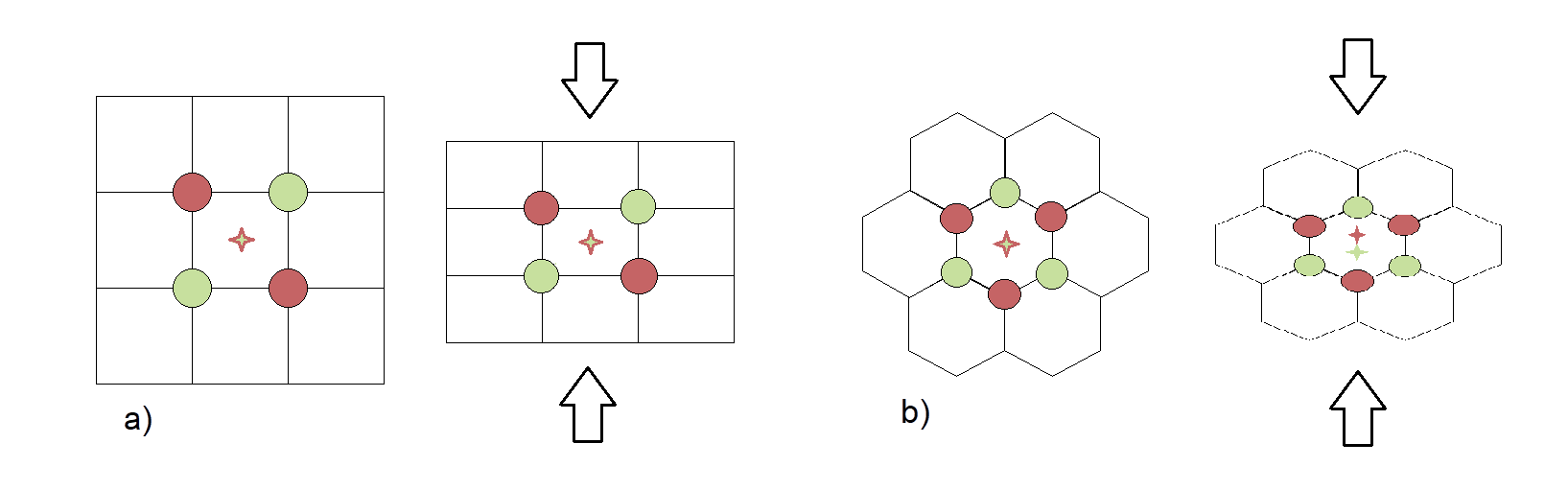## Piezoelectricity

When an external force is applied to a crystal, we generally expect the positions of the atoms in the lattice to change in response to mechanical stress. If this response leads to a change in polarization as well, the material is called piezoelectric.

Piezoelectricity, as well as pyro- and ferroelectricity, is a material property that is strongly tied to crystal symmetries. As will be discussed in detail, only crystals which do not exhibit inversion symmetry are potentially piezoelectric. Of all 32 point groups, 21 are noncentrosymmetric, and of those 20 point groups show piezoelectricity (only point group 432 is an exception). Those piezoelectric point groups can be further divided into ten polar and ten nonpolar ones. The former exhibit spontaneous polarization and are called pyroelectric. For some of the pyroelectric groups, this spontaneous polarization can be reversed with an outer electric field, and those materials are then called ferroelectrics.

Simply put: All ferroelectrics in their polar phase are pyro- and thus piezoelectric. However, not every piezoelectric shows pyro- or ferroelectricity.Fig. 1 Relation between piezo-, pyro- and ferroelectric point groups 
11 centrosymmetric point groups: 1, 2/m, mmm, 4/m, 4/mmm, 3, 3m, 6/m, 6/mmm, m3, and m3m
21 noncentrosymmetric point groups:
exception: 432
20 piezoelectric:
10 polar: 1, 2, m, mm2, 3, 3m, 4, 4mm, 6, 6mm
10 nonpolar: 222, 4, 422, 42m, 32, 6, 622, 62m, 23, 43m

### Inversion Symmetry

In order to understand why symmetry plays such an important role for piezoelectricity, we can compare the response to an external force of a crystal structure with inversion symmetry and one without.Fig. 2 External force acting on an ionic crystal with (a) and without (b) inversion symmetry 

In Fig. 2 (a), a plane of a cubic crystal like NaCl is shown. If no force is applied, the centers of charge of the positive and negative ions overlap at the center of unit cell of the lattice; the overall polarization $\vec{P}$ of this system zero. If a force is applied, the atoms are pressed together horizontally, but the centers of charge still won't move due to the symmetry of the charged atoms at their respective corners; any displacement of a charge in downward direction in the upper row is mirrored by a displacement in upward direction in the lower row. For an inversion-symmetric crystal, the polarization remains zero, even under stress.

In contrast, consider the hexagonal crystal without inversion symmetry in Fig. 2 (b); this could represent a plane of Wurtzite ($\beta$-ZnS). The centers of charge of the positive and negative ions overlap again at the center of each cell without an external force, so the overall polarization in the crystal is zero. However, if a force is applied now, the centers of charge of the negative atoms and the positive atoms are suddenly shifted against each other due to their triangular symmetry. For each unit cell, a polarization appears, and thus the overall polarization of the non-inversion-symmetric crystal under stress is no longer zero. At the upper and lower edges of the material, negative resp. positive charges will accumulate, and a voltage can suddenly be measured.

### Piezoelectricity Tensor

The considerations from above can also be described mathematically. For no outer electric field, the relation between polarization and stress (direct piezoelectric effect) can be written as: \begin{equation} \vec{P} = \pmb{d} \pmb{\sigma} \end{equation}

with $\vec{P}$ the polarization vector, $\pmb{\sigma}$ the second-rank stress tensor, and $\pmb{d}$ the third-rank tensor describing the direct piezoelectric effect. For an inversion-symmetric system, all entries of an odd rank tensor have to vanish (which is shown for pyroelectricity in the lecture notes and can be proven the same way for higher odd-rank tensors as well). This means no properties described by an odd rank tensor can be observed in an inversion-symmetric system. Such a crystal exhibits neither pyro- (rank one) nor piezoelectricity (rank three).

### Everyday applications

A technical application to piezoelectricity are quartz crystal oscillators as used in quartz clocks. Here, the piezoelectric quartz is formed like a miniature turning fork and an AC field leads to a periodic structural change of the crystal (this is known as the inverse or converse piezoelectric effect). These quartz forks usually have high Eigenfrequencies, which is convenient, as the highest audible frequency for the human ear is at about 20 kHz, so there is no possibility of hearing the wrist watch work. The quartz is specifically designed to oscillate with a frequency of $2^{15}$Hz (the first power of 2 above 20k). With 15 consecutive flipflops, the signal is then reduced to a 1 Hz signal feeding the second hand of the watch.

Another application are BBQ lighters; the (normally hard-to-push) button usually tightens a spring mechanism, which strikes a hammer on the crystal hard enough to create high voltage for a short amount of time. The spark, combined with some flammable gas, is then used to light the fire.

1. R. K. Pandey, Fundamentals of Electroceramics: Materials, Devices, and Applications

2. H. Ohsato, Origin of Piezoelectricity on Langasite, Materials Science and Technology, https://www.yumpu.com/en/document/read/13289580/2-origin-of-piezoelectricity-on-langasite-cdn-intech, (2012)

3. Ch. Weissmantel, C. Hamann, Grundlagen der Festkörperphysik, (1979)